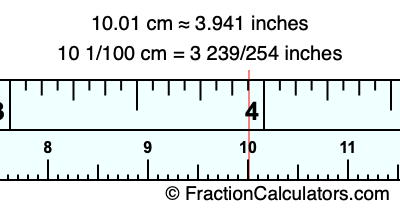10.01 cm in inchesHere is how to convert 10.01 cm to inches. We will give you the fractional answer, the decimal answer, and illustrate the answer on our tape measure.

One centimeter is equal to 50/127 inches, and 10.01 as a fraction is 10 1/100. Therefore, to get the fractional answer to 10.01 cm in inches, we multiply 10 1/100 by 50/127 and simplify if necessary.

cm × 50/127 = inches
10 1/100 × 50/127 = inches
10 1/100 × 50/127 = 3 239/254
10 1/100 cm = 3 239/254 inches
10.01 cm = 3 239/254 inches

As promised above, we also have the answer to 10.01 cm in inches in decimal form. Although the fractional answer above is exact, the decimal answer may be rounded if necessary:

3 239/254 ≈ 3.94094488188976
3 239/254 ≈ 3.941
10.01 cm ≈ 3.941 inches

Our image below shows 10.01 cm on a tape measure. The top row of the tape measure is inches, and the bottom row is centimeters.As you can see, we drew a red line where 10.01 cm and 3.941 inches meet on the measuring tape.

Centimeters to Inches Calculator
Here you can convert another length in centimeters to inches.

Convert  cm to inches.

What is 10.02 cm in inches?
Here is another inches to centimeters calculation we did for you.